GACE Middle Grades Mathematics (013): Practice & Study Guide Final Exam

Free Practice Test Instructions:

Choose your answer to the question and click 'Continue' to see how you did. Then click 'Next Question' to answer the next question. When you have completed the free practice test, click 'View Results' to see your results. Good luck!

Answered 0 of 15

Question 2 2. Triangle ABC is reflected across the x axis to form triangle A'B'C' and then rotated counterclockwise about point C' by 270 degrees. Which of the following figures shows the resulting triangle?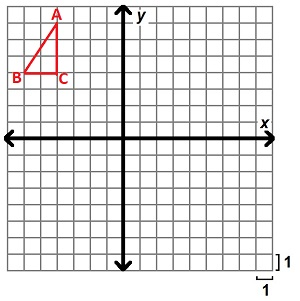Question 5 5. Which of the following is an equation of the quadratic function shown in the xy-plane?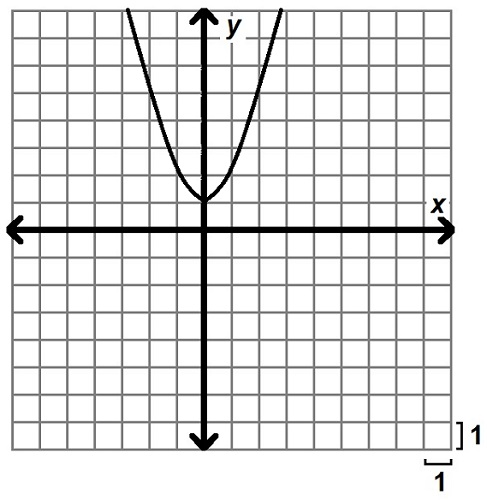Question 6 6. If lines l and p are parallel and x = 4y, what is the value of x?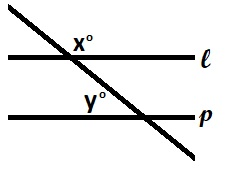Question 7 7. The large block shown is made through the stacking of identical blocks that have which of the following dimensions?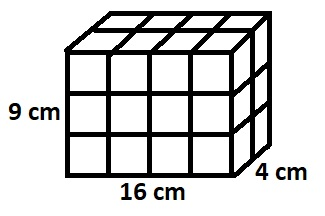Question 8 8. The table shows the number of hot dogs eaten by competitors in a hot dog eating competition. What is the median number of hot dogs eaten?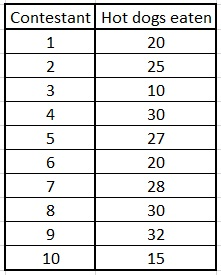Question 11 11. The graph shows the distribution of visits by animal type for a certain veterinary practice over the course of one year. If the office sees 15 cows in a given month, approximately how many dogs are predicted to be seen in the same month?Question 15 15. Which of the following is true about the data in the table?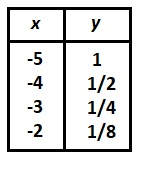Are you a student or a teacher?

GACE Middle Grades Mathematics (013): Practice & Study Guide Final Free Practice Test Instructions

Choose your answer to the question and click 'Continue' to see how you did. Then click 'Next Question' to answer the next question. When you have completed the free practice test, click 'View Results' to see your results. Good luck!

Support